Comparing fractions

Chapter 7 Class 6 Fractions
Concept wise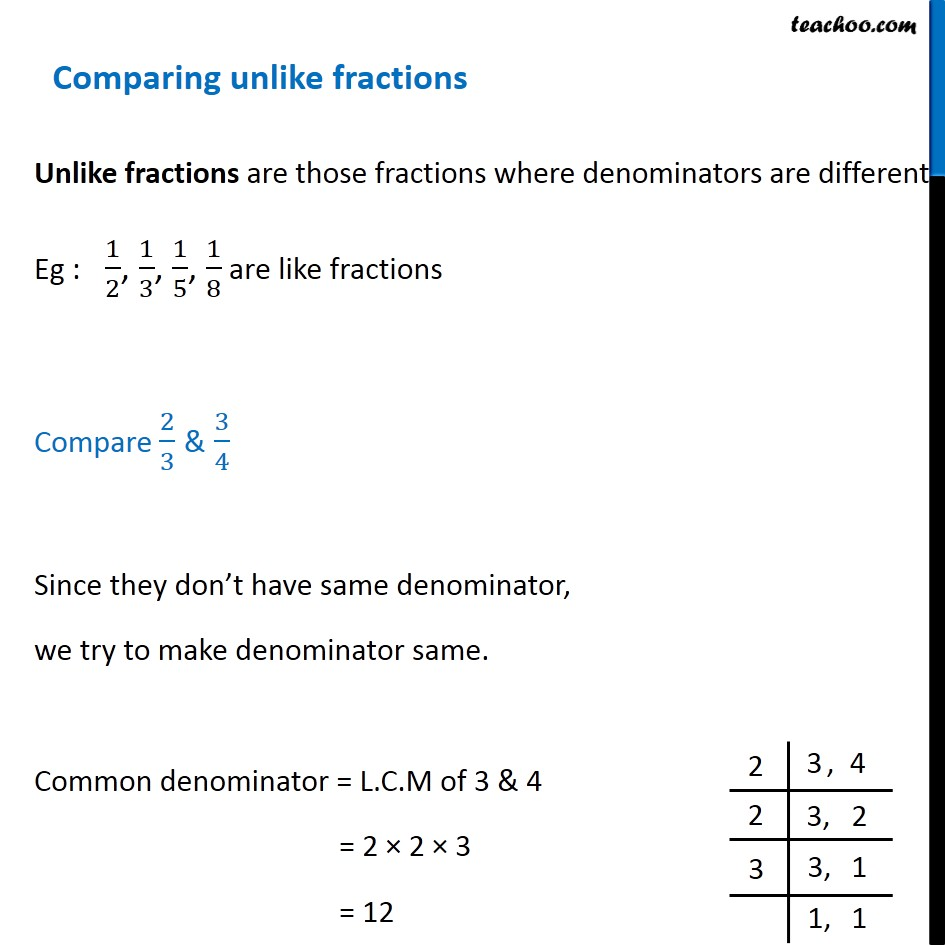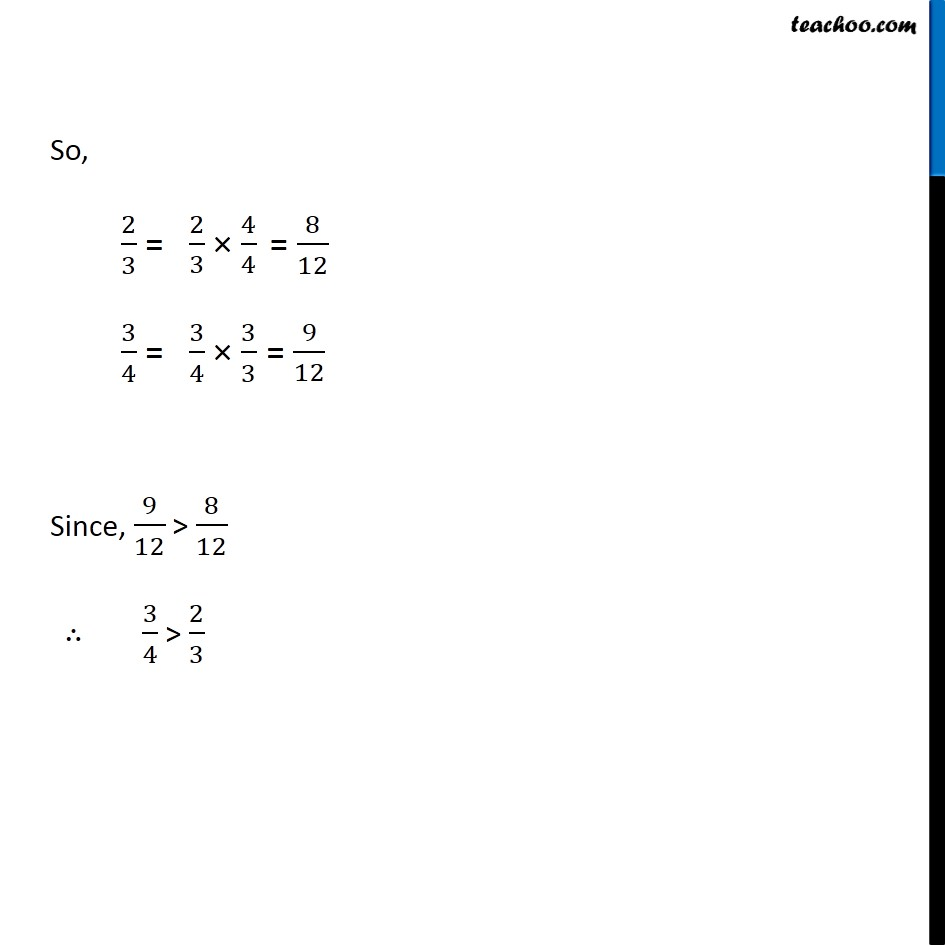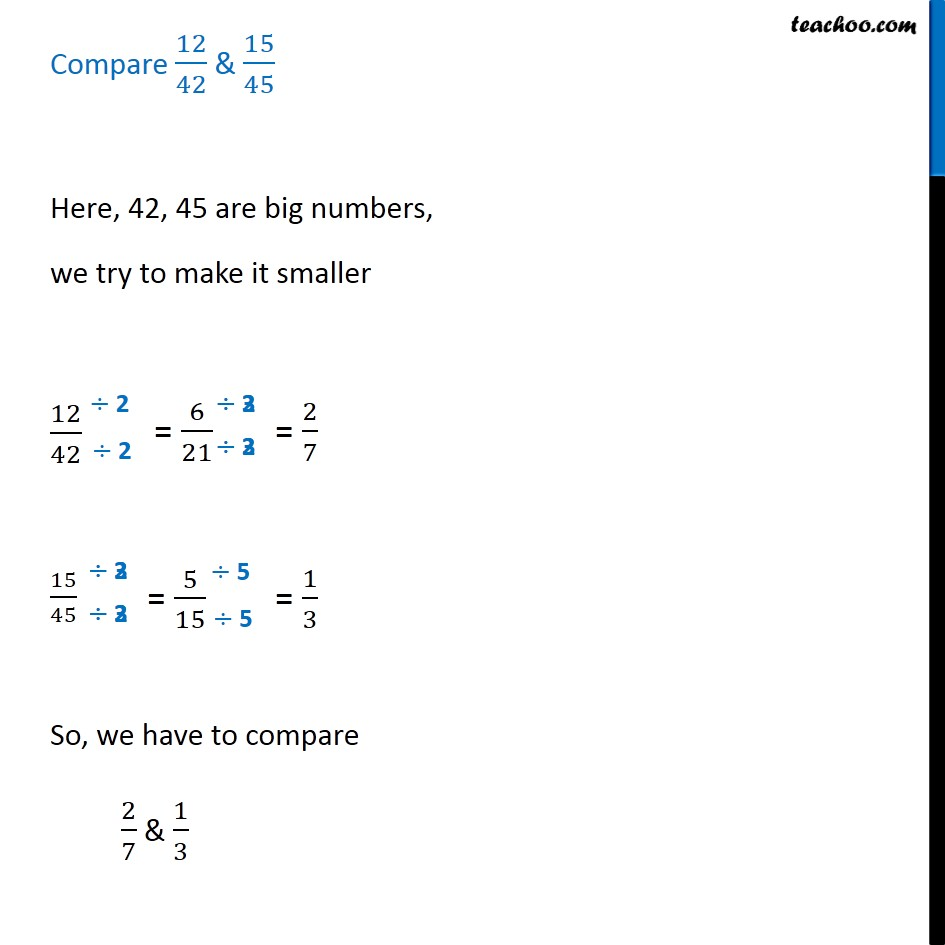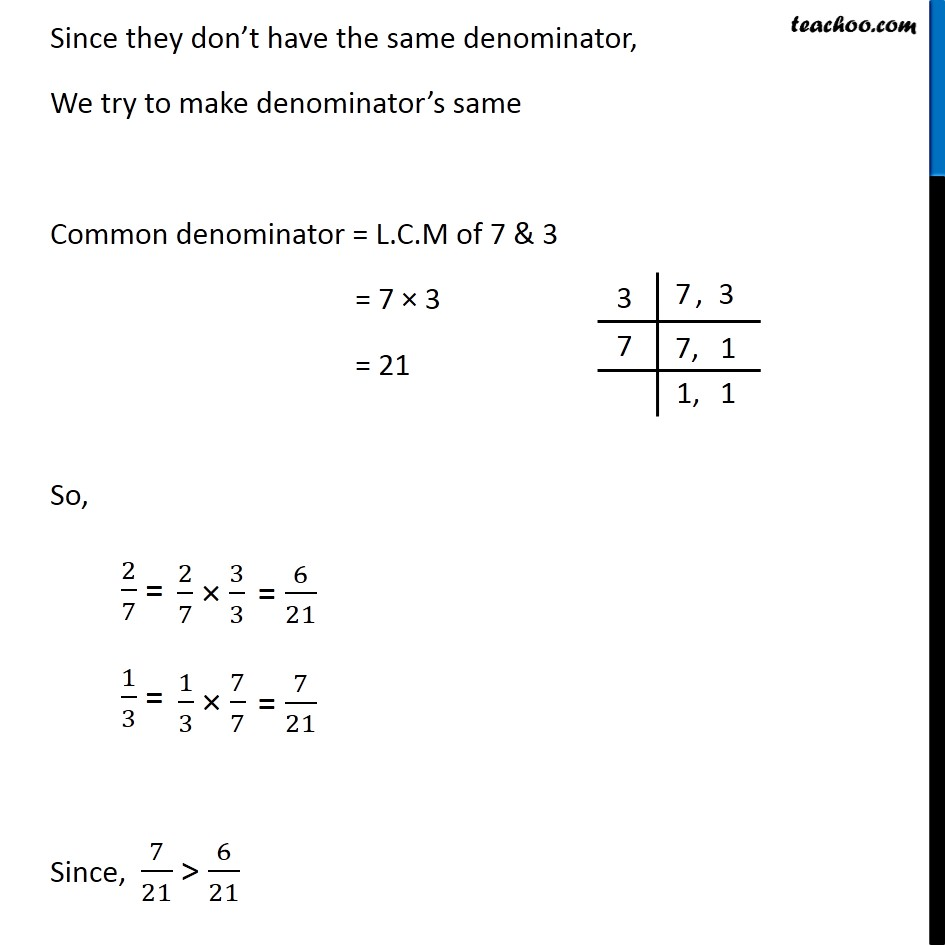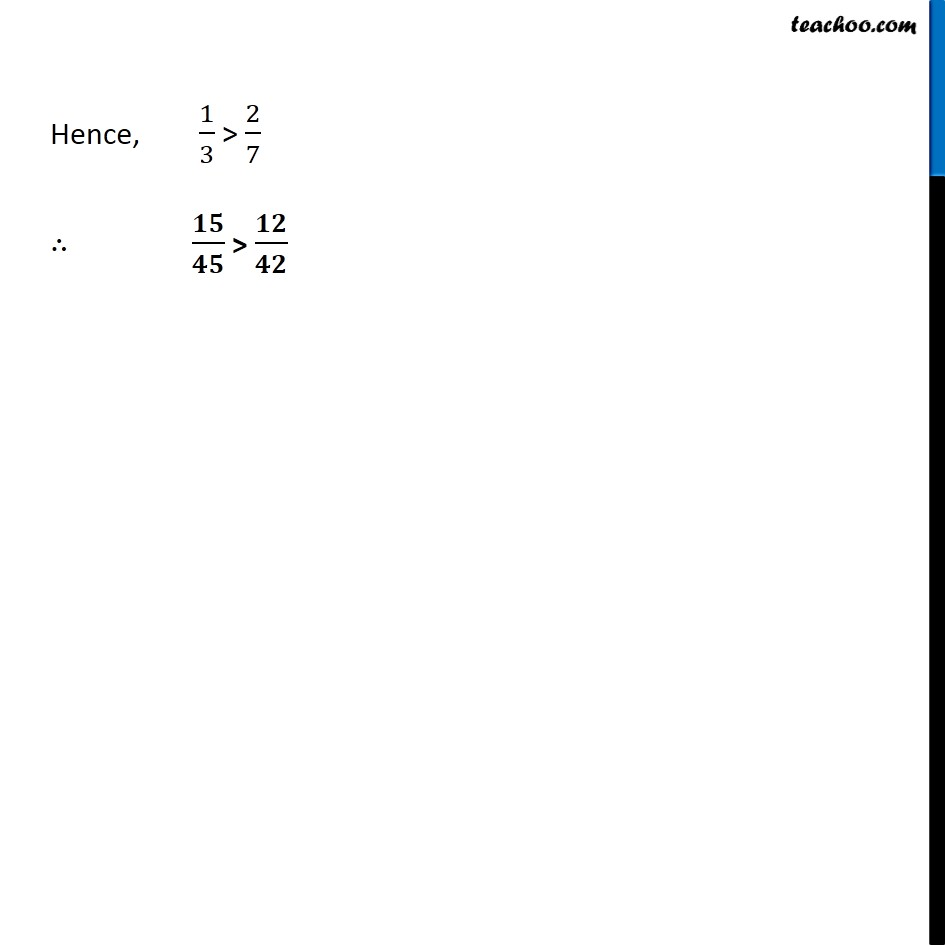Learn in your speed, with individual attention - Teachoo Maths 1-on-1 Class

### Transcript

Comparing unlike fractionsUnlike fractions are those fractions where denominators are different. Eg : 1/2, 1/3, 1/5, 1/8 are like fractions Compare 2/3 & 3/4 Since they don’t have same denominator, we try to make denominator same. Common denominator = L.C.M of 3 & 4 = 2 × 2 × 3 = 12 2 3, 4 2 3, 2 3 3, 1 1, 1 So, 2/3 = 2/3 × 4/4= 8/12 3/4 =3/4 × 3/3= 9/12 Since, 9/12 > 8/12 ∴ 3/4 > 2/3 Compare 12/42 & 15/45 Here, 42, 45 are big numbers, we try to make it smaller 12/42 ÷ 2 ÷ 2 = 6/21 3 = 2/7 15/45 ÷ 3 ÷ 3 = 5/15 5 5 = 1/3 So, we have to compare 2/7 & 1/3 Since they don’t have the same denominator, We try to make denominator’s same Common denominator = L.C.M of 7 & 3 = 7 × 3 = 21 So, 2/7 = 2/7 × 3/3× = 6/21 1/3 = 1/3 x 7/7 = 6/21 since, 7/21 > 6/21 Hence, 1/3 > 2/7 ∴ 𝟏𝟓/𝟒𝟓 > 𝟏𝟐/𝟒𝟐